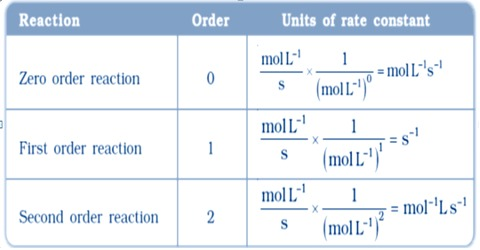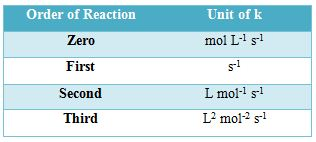Chemistry

# Units of Rate ConstantsUnits of Rate Constants

The units of rate constants are derived from the rate equation and depend on the order of the reaction. In chemical kinetics a reaction rate constant or reaction rate coefficient, k, quantifies the rate of a chemical reaction. Thus considering time in second the units of ‘k’ are given in the Table below:

Table: Units of the rate constantsIn some reactions, the rate is apparently independent of the reactant concentration. The rates of these zero-order reactions do not vary with increasing nor decreasing reactants concentrations. A first order reaction depends on the concentration of only one reactant (a unimolecular reaction). Other reactants can be present, but each will be zero order. A second order reaction is a type of chemical reaction that depends on the concentrations of one second order reactant or on two first order reactants.